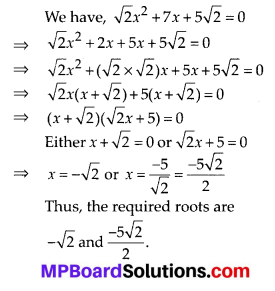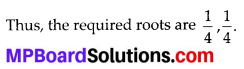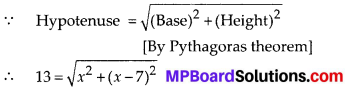In this article, we will share MP Board Class 10th Maths Book Solutions Chapter 4 Quadratic Equations Ex 4.2 Pdf, These solutions are solved subject experts from the latest edition books.

## MP Board Class 10th Maths Solutions Chapter 4 Quadratic Equations Ex 4.2

Question 1.
Find the roots of the following quadratic equations by factorisation:
(i) x2 – 3x – 10 = 0
(ii) 2x2 + x – 6 = 0
(iii) $$\sqrt{2} x^{2}+7 x+5 \sqrt{2}$$ = 0
(iv) 2x2 – x + $$\frac{1}{8}$$ = 0
(v) 100x2 – 20x + 1 =0
Solution:
(i) We have, x2 – 3x – 10 = 0
⇒ x2 – 5x + 2x – 10 = 0
⇒ x(x – 5) + 2(x – 5) = 0 (x – 5)(x + 2) = 0
Either x – 5 = 0 or x + 2 = 0
x = 5 or x = – 2
Thus, the required roots are 5 and -2.

(ii) We have, 2x2 + x – 6 = 0
⇒ 2x2 + 4x – 3x – 6 = 0
⇒ 2x(x + 2) – 3(x + 2) = 0
⇒ (x + 2)(2x – 3) = 0
Either x + 2 = 0 or 2x – 3 = 0
⇒ x = -2 or x = $$\frac{3}{2}$$
Thus, the required roots are -2 and $$\frac{3}{2}$$

(iii)(iv) We have, 2x2 – x + $$\frac{1}{8}$$ = 0
⇒ 16x2 – 8x + 1 = 0
⇒ 16x2 – 4x – 4x + 1 = 0
⇒ 4x(4x – 1) -1(4x – 1) = 0
⇒ 4x – 1 = 0
⇒ x = $$\frac{1}{4}$$(v) We have 100x2 – 20x + 1 = 0
⇒ 100x2 – 10x – 10x + 1 = 0
⇒ 10x(10x – 1) -1(10x – 1) = 0
⇒ (10x – 1)(10x – 1) = 0
⇒ (10x – 1) = 0
⇒ x = $$\frac{1}{10}$$
Thus the required roots are $$\frac{1}{10}, \frac{1}{10}$$Question 2.
Solve the problems:
(i) John and Jivanti together have 45 marbles. Both of them lost 5 marbles each, and the product of the number of marbles they now have is 124. We would like to find out how many marbles they had to start with.
(ii) A cottage industry produces a certain number of toys in a day. The cost of production of each toy (in rupees) was found to be 55 minus the number of toys produced in a day. On a particular day, the total cost of production was ₹ 750. We would like to find out the number of toys produced on that day.
Solution:
(i) Let John had x marbles and Jivanti had (45 – x) marbles.
When both of them lost 5 marbles then equation becomes (x – 5) × (45 – x – 5) = 124
⇒ (x – 5) × (40 – x) = 124
⇒ x2 – 45x + 324 = 0
⇒ x2 – 9x – 36x + 324 = 0
⇒ x(x – 9) – 36(x – 9) = 0
⇒ (x – 9)(x – 36) = 0
Either x – 9 = 0 or x – 36 = 0
Thus, x = 9 or x = 36
∴ If John had 9 marbles, then Jivanti had 45 – 9 = 36 marbles.
If John had 36 marbles, then Jivanti had 45 – 36 = 9 marbles.

(ii) Let the number of toys produced in a day be x.
Then cost of 1 toy = $$\frac{750}{x}$$
⇒ $$\frac{750}{x}$$ = 55 – x
⇒ 750 = 55x – x2
⇒ x2 – 55x + 750 = 0
⇒ x2 – 30x – 25x + 750 = 0
⇒ x(x – 30) – 25(x – 30) = 0
⇒ (x – 30)(x – 25) = 0
Either x – 30 = 0 or x – 25 = 0
x = 30 or x = 25Question 3.
Find two numbers whose sum is 27 and product is 182.
Solution:
Let one of the numbers be x.
∴ Other number = 27 – x
According to the condition,
x(27 – x) = 182
⇒ 21x – x2 = 182
⇒ x2 – 27x + 182 = 0
⇒ x2 – 13x – 14x +182 = 0
⇒ x(x – 13) – 14(x – 13) = 0
⇒ (x – 13)(x – 14) = 0
Either x – 13 = 0 or x -14 = 0
⇒ x = 13 or x = 14
Thus, the required numbers are 13 and 14.

Question 4.
Find two consecutive positive integers, sum of whose squares is 365.
Solution:
Let the two consecutive positive integers be x and (x + 1).
Since, the sum of the square of the numbers is 365.
∴ x2 + (x + 1)2 = 365
⇒ x2 + (x2 + 2x + 1) = 365
⇒ x2 + x2 + 2x + 1 = 365
⇒ 2x2 + 2x + 1 – 365 = 0
⇒ 2x2 + 2x – 364 = 0
⇒ x2 + x – 182 = 0
⇒ x2 + 14x – 13x – 182 = 0
⇒ x(x + 14) – 13(x + 14) = 0
⇒ (x + 14)(x -13) = 0
Either x + 14 = 0 or x – 13 = 0
⇒ x = -14 or x = 13
Since, x has to be a positive integer
⇒ x = -14 is rejected.
∴ x = 13 ⇒ x + 1 = 13 + 1 = 14
Thus, the required consecutive positive integers are 13 and 14.Question 5.
The altitude of a right triangle is 7 cm less than its base. If the hypotenuse is 13 cm, find the other two sides.
Solution:
Let the base of the given right triangle be x cm.
∴ Its height = (x – 7) cmSquaring both sides, we get
169 = x2 + (x – 7)2
⇒ 169 = x2 + x2 – 14x + 49
⇒ 2x2 – 14x + 49 – 169 = 0
⇒ 2x2 – 14x – 120 = 0
⇒ x2 – 7x- 60 = 0
⇒ x2 – 12x + 5x – 60 = 0
⇒ x(x – 12) + 5(x – 12) = 0
⇒ (x – 12)(x + 5) = 0
Either x – 12 = 0 or x + 5 = 0
⇒ x = 12 or x = -5
But the sides of a triangle can never be negative
⇒ x = -5 is rejected.
∴ x = 12
∴ Length of base = 12 cm
⇒ Length of altitude = (12 – 7)cm = 5 cm
Thus, the required base = 12 cm and altitude = 5 cmQuestion 6.
A cottage industry produces a certain number of pottery articles in a day. It was observed on a particular day that the cost of production of each article (in rupees) was 3 more than twice the number of articles produced on that day. If the total cost of production on that day was ₹ 90, find the number of articles produced and the cost of each article.
Solution:
Let the number of articles produced in a day = x
∴ Cost of production of each article = ₹ (2x + 3)
According to the condition,
Total cost = ₹ 90
⇒ x × (2x + 3) = 90
⇒ 2x2 + 3x = 90
⇒ 2x2 + 3x – 90 = 0
⇒ 2x2 – 12x + 15x – 90 = 0
⇒ 2x(x – 6) + 15(x – 6) = 0
⇒ (x – 6)(2x + 15) = 0
Either x – 6 = 0 or 2x + 15 = 0
⇒ x= 6 or x = $$\frac{-15}{2}$$
But the number of articles produced can never be negative.
⇒ x = $$\frac{-15}{2}$$ is rejected
∴ Cost of production of each article = ₹ (2 × 6 + 3) = ₹ 15
Thus, the required number of articles produced is 6 and the cost of each article is ₹ 15.